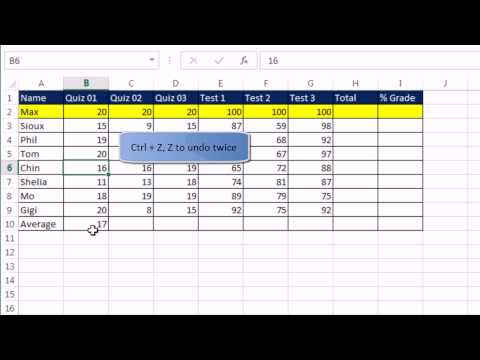### Excel Introduction Video# Excel Introduction Video

## 31 Video Interactions

1. 00:00:27

Which Microsoft Office product is this video about?

• Microsoft Office

• Microsoft Access

• Microsoft Excel

2. 00:00:42

Which version of Microsoft Excel do we use?

• 2007

• 2010

• 2013

• 2020

3. 00:01:00

What do we call an Excel file?

• document

• workbook

• database

• powerpoint

4. 00:02:10

What is represented by letters in an Excel spreadsheet?

• Rows

• Columns

5. 00:02:12

What is represented by numbers in an Excel spreadsheet?

• Rows

• Columns

6. 00:02:24

What is the intersection of a row and a column called?

• cell

• formula

• grid

7. 00:02:46

All the cells together in excel are a _________.

• worksheet

• file

• document

8. 00:03:16

Can I rename sheets in Excel?

• yes

• no

9. 00:04:45

Can I have more than one worksheet in Excel?

• yes

• no

10. 00:05:16

What button enters data in the cell and then moves to the next cell?

• Enter

• Tab

• Space Bar

11. 00:06:07

What is the square in the bottom right of the active cell?

• fill handle

• pot handle

• fridge handle

12. 00:06:31

What is the shortcut to UNDO an edit in Excel?

• control H

• control s

• control z

13. 00:07:54

In the default setting of Excel numbers are always aligned to the ______.

• left

• right

14. 00:10:36

What is the first way he mentioned to select the entire table?

• Click and drag

• hit enter 4 times

• Shift control F

15. 00:11:51

Can I format one row differently than the rest?

• Yes

• no

16. 00:12:04

Text in 1st row of a table of data that indicate what sort of data can go in each column are called:

• Columns and fields

• fountains

17. 00:13:11

What is a good way to show that one part of the table is the heading?

• Put it in smaller text

• Make the background a different color

• Leave it blank

18. 00:15:08

What do we use to calculate values in Excel?

• droplets

• columns

• formulas

19. 00:16:01

In this formula, he didn't point it out, but what did the formula start with?  EVERY FORMULA STARTS WITH THIS!!!!

• =

• +

• -

20. 00:16:25

• yes

• no

21. 00:18:54

What puts the cell in edit mode so I can view the formula?

• F4

• F2

• F6

22. 00:19:27

If you need to change a number in Excel, does the formula automatically update?

• No

• Yes

23. 00:20:30

GOLDEN RULE:  Refer to the data not as numbers, but as ____________.

• letters

• astroids

• cell references

24. 00:22:25

When you copy the formulas, do the cell references change?

• Yes

• No

25. 00:24:57

Again, what do I start EVERY FORMULA WITH?

• An equal sign

• A subtraction sign

26. 00:27:13

Which symbol did he use for division?

• /

• ?

• +

27. 00:29:54

We use a \$ to tell the formula that number is absolute and the reference will not change.

• false

• true

28. 00:31:17

What key should you use to delete content?

• tab

• enter

• delete

29. 00:33:58

Can we format numbers to look differently?  Such as, if I type in .86, can I turn it into 86% without retyping it?

• Yes

• No

30. 00:34:46

How do I see my numbers unformatted?

• Apply General formatting

• Apply percentage formatting

• Apply currency formatting

31. 00:35:44

What is the shortcut to print?

• Control P

• Control S

• Control G

Click here to preview, use, or make a copy of this interactive video lesson plan (bulb).